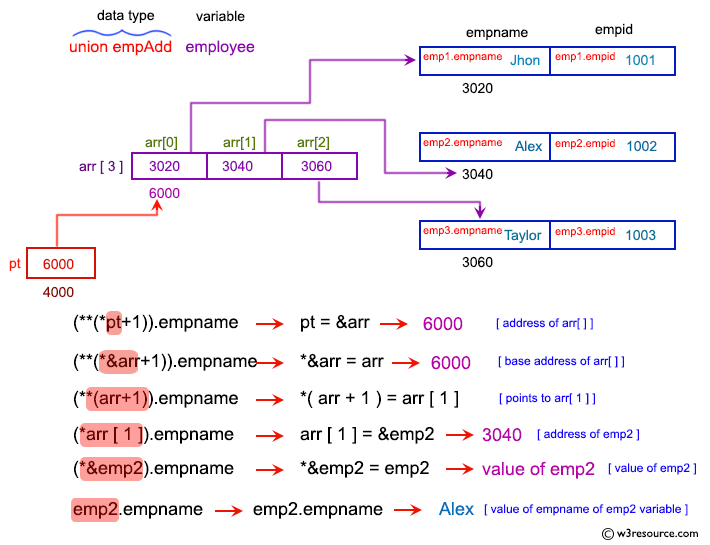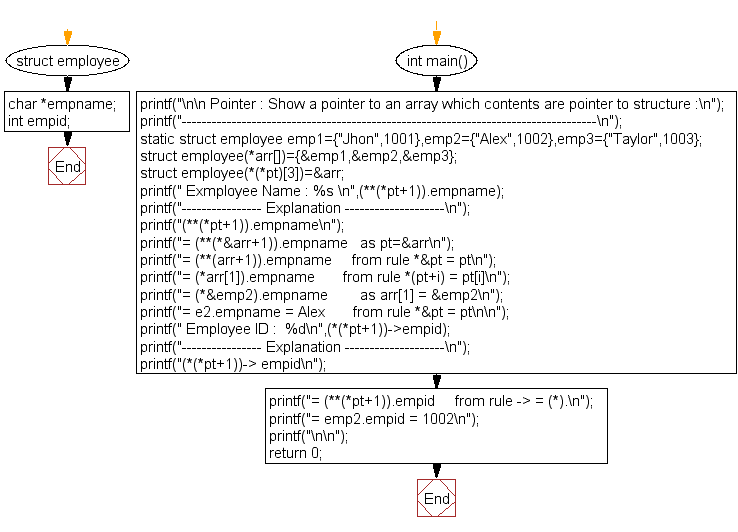﻿ C Program: Array pointer, contents pointer to structure# C Exercises: Show a pointer to an array which contents are pointer to structure

## C Pointer : Exercise-20 with Solution

Write a program in C to show a pointer to an array which contents are pointer to structure.

Pictorial Presentation:Sample Solution:

C Code:

``````#include <stdio.h>
struct employee
{
char *empname;
int empid;
};

int main()
{
printf("\n\n Pointer : Show a pointer to an array which contents are pointer to structure :\n");
printf("-----------------------------------------------------------------------------------\n");

static struct employee emp1={"Jhon",1001},emp2={"Alex",1002},emp3={"Taylor",1003};
struct employee(*arr[])={&emp1,&emp2,&emp3};
struct employee(*(*pt))=&arr;

printf(" Exmployee Name : %s \n",(**(*pt+1)).empname);
printf("---------------- Explanation --------------------\n");
printf("(**(*pt+1)).empname\n");
printf("= (**(*&arr+1)).empname   as pt=&arr\n");
printf("= (**(arr+1)).empname     from rule *&pt = pt\n");
printf("= (*arr).empname       from rule *(pt+i) = pt[i]\n");
printf("= (*&emp2).empname        as arr = &emp2\n");
printf("= emp2.empname = Alex       from rule *&pt = pt\n\n");
printf(" Employee ID :  %d\n",(*(*pt+1))->empid);
printf("---------------- Explanation --------------------\n");
printf("(*(*pt+1))-> empid\n");
printf("= (**(*pt+1)).empid     from rule -> = (*).\n");
printf("= emp2.empid = 1002\n");
printf("\n\n");
return 0;
}
```
```

Sample Output:

``` Pointer : Show a pointer to an array which contents are pointer to structure :
-----------------------------------------------------------------------------------
Exmployee Name : Alex
---------------- Explanation --------------------
(**(*pt+1)).empname
= (**(*&arr+1)).empname   as pt=&arr
= (**(arr+1)).empname     from rule *&pt = pt
= (*arr).empname       from rule *(pt+i) = pt[i]
= (*&emp2).empname        as arr = &emp2
= emp2.empname = Alex       from rule *&pt = pt

Employee ID :  1002
---------------- Explanation --------------------
(*(*pt+1))-> empid
= (**(*pt+1)).empid     from rule -> = (*).
= emp2.empid = 1002
```

Flowchart:C Programming Code Editor:

Have another way to solve this solution? Contribute your code (and comments) through Disqus.

What is the difficulty level of this exercise?

Test your Programming skills with w3resource's quiz.

﻿

## C Programming: Tips of the Day

How to format strings using printf() to get equal length in the output?

You can specify a width on string fields, e.g.

`printf("%-20s", "initialization..."); `

And then whatever's printed with that field will be blank-padded to the width you indicate.

The - left-justifies your text in that field.

Ref : https://bit.ly/34DMOc3